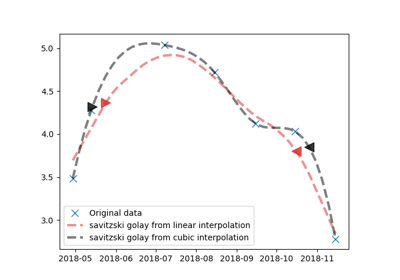# get_phenology_metrics¶

`museopheno.time_series.``get_phenology_metrics`(X, sos=0.2, eos=0.8, min_from_year=False)[source]

Return indices of phenology metrics (start of season and end of season).

Parameters
• X (array) – array can be two dimensions (a line per sample)

• sos (float, optional (default=0.2)) – Percentage (0.2 for 20%) of the season amplitude

• eos (float, optional (default=0.8)) – Percentage (0.8 for 80%) of the season amplitude

• min_from_year (bool, optional (default=False)) – If True, metrics thresolds will be computed using the min from the year If False, metrics thresolds will be computed using the min from the season (start or end).

Returns

• Array with as many lines as samples.

• First column is SOS index, second is EOS index.

## Examples using `museopheno.time_series.get_phenology_metrics`¶